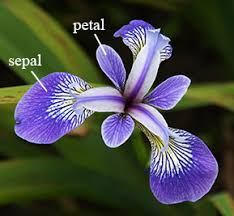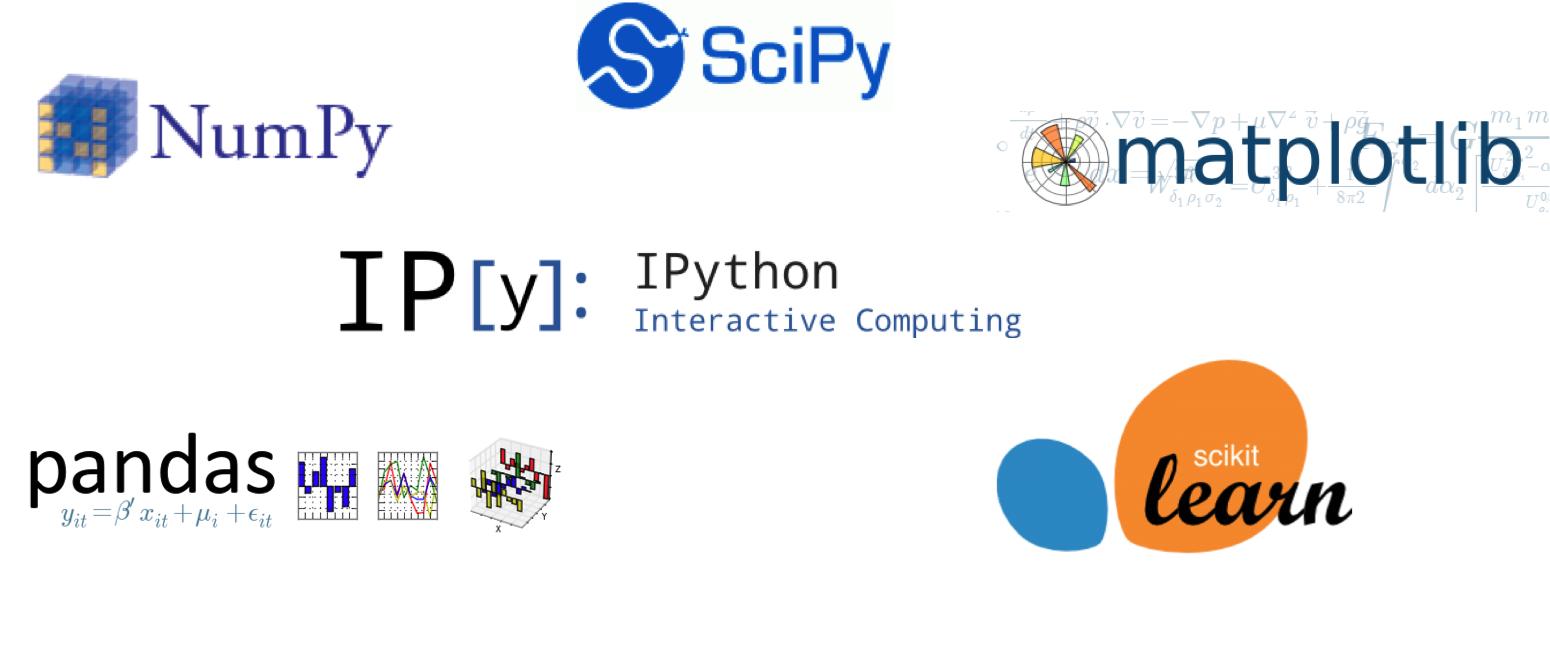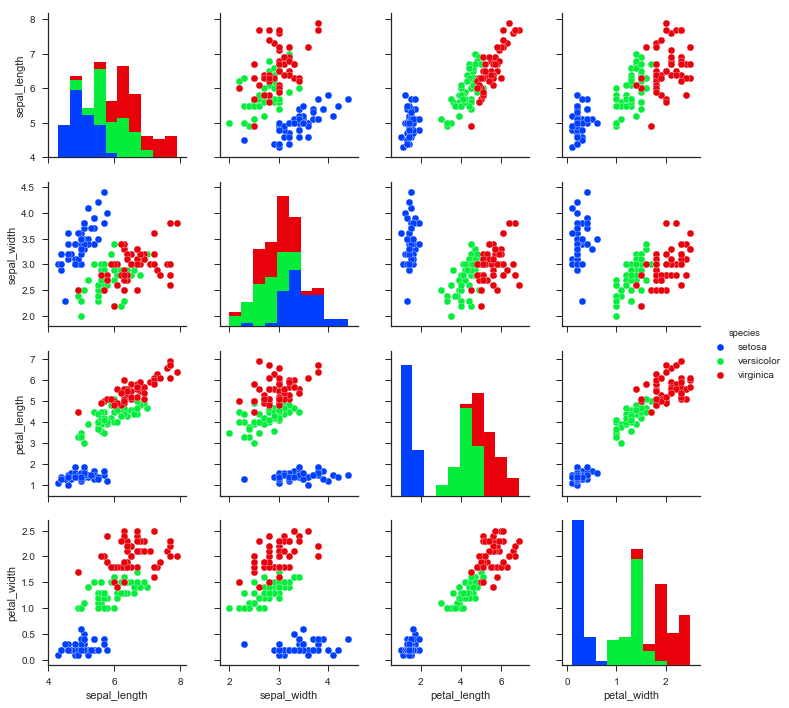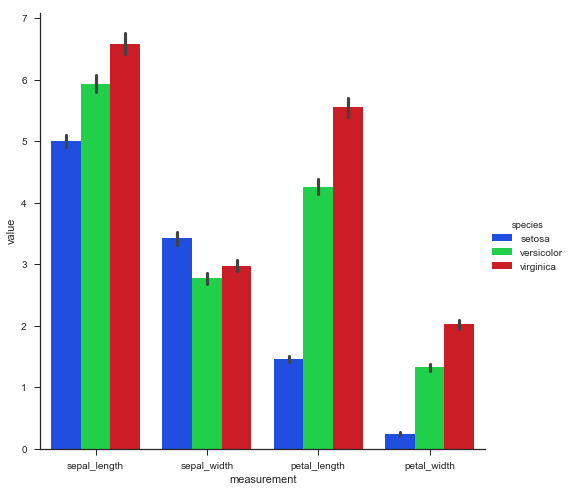# Machine Learning on Iris

A post on the use of Machine Learning to classify the species of the iris flower

Diwash Shrestha https://diwashrestha.com.np
09-18-2017

In this blog, we will use some machine learning concept with help of ScikitLearn a Machine Learning Package and Iris dataset which can be loaded from sci-kit learn. we will use numpy to work on the Iris dataset and Matplotlib for Visualization. Iris data set is a multivariate data set introduced by the British statistician and biologist Ronald Fisher in his 1936 paper. The use of multiple measurements in taxonomic problems as an example of linear discriminant analysis. The data set consists of 50 samples from each of three species of Iris:

• Iris setosa
• Iris virginica
• Iris versicolorThere are Four features or column about the flowe r.

• Sepal length(cm)
• Sepal Width(cm)
• Petal Length(cm)
• Petal Width(cm)Iris datasets are the basic Machine Learning data. The objective of this post is to find the species of Iris flower of test data using the trained model. we are using the Sklearn python package’s Decision tree.

## Import Library and moduleFirst, we will import the required library and module in the python console. In this machine learning we will use:

• Numpy: which provides support for more efficient numerical computation

• Pandas: a convenient library that supports data frames.

• Matplotlib &Seaborne: for Visualization

• ScikitLearn: Machine learning tools


import numpy as np
import matplotlib.pyplot as plt
import seaborn as sns
import pandas as pd
from sklearn import tree
from sklearn.model_selection import train_test_split
from sklearn.metrics import accuracy_score
from sklearn.externals import joblib

Now, we will load the iris data from the seaborne’s builtin dataset and print first 5 rows as follow:


print(iris.head())

sepal_length  sepal_width  petal_length  petal_width species
5.1          3.5           1.4          0.2  setosa
4.9          3.0           1.4          0.2  setosa
4.7          3.2           1.3          0.2  setosa
4.6          3.1           1.5          0.2  setosa
5.0          3.6           1.4          0.2  setosa

Lets look at the data


print (iris.shape)

#(150, 5)

we have 150 samples and 5 features, including our target feature. we can easily print some summary statistics.


print(iris.describe())

sepal_length  sepal_width  petal_length  petal_width
count    150.000000   150.000000    150.000000   150.000000
mean       5.843333     3.057333      3.758000     1.199333
std        0.828066     0.435866      1.765298     0.762238
min        4.300000     2.000000      1.000000     0.100000
25%        5.100000     2.800000      1.600000     0.300000
50%        5.800000     3.000000      4.350000     1.300000
75%        6.400000     3.300000      5.100000     1.800000
max        7.900000     4.400000      6.900000     2.500000

The list of the features are :

• sepal length
• sepal width
• petal length
• petal width

## Split data into training and test sets

we split the data into training and test sets at the beginning of modelling workflow. Splitting is crucial for getting a realistic estimate of the model’s performance.

First, let’s separate our target (y) features from our input (X) features:


y = iris.species
X = iris.drop('species',axis=1)

Now we use the Scikit learn train_test_split function:


from sklearn.model_selection import train_test_split
X_train, X_test, y_train, y_test = train_test_split(X, y,
test_size=0.3,
random_state=100,
stratify=y)

we’ll set aside 30% of the data as a test set for evaluating the model. we also set an arbitrary “random state” so that the program can reproduce our results.

## Visualization

Now we will plot the graph to understand the features and the species in data.we are using seaborne and matplotlib to make these graph plots.


sns.set(style="ticks")
sns.pairplot(iris, hue="species",palette="bright")
plt.show()The above graph is scatterplot which is plotted between four features of iris in 12 different plots. In the above picture, we can see the samples formed clusters according to their species.

In next graph, we will plot the 4 features of 3 iris species in barplot:


piris = pd.melt(iris, "species", var_name="measurement") sns.factorplot(x="measurement", y="value", hue="species", data=piris, size=7, kind="bar",palette="bright") plt.show()
print(piris.head())

species   measurement  value
setosa  sepal_length    5.1
setosa  sepal_length    4.9
setosa  sepal_length    4.7
setosa  sepal_length    4.6
setosa  sepal_length    5.0In the above code, we made a new variable piris to make the visualization easier. This picture shows how three species of iris differ on the basis of the four features.

## Decision tree

Decision tree algorithm is a simple supervised learning algorithm which is used in regression and classification problems. we will make Decision Tree classifier and fit training data (X_train and y_train) to train the model.


clf = tree.DecisionTreeClassifier()
clf.fit(X_train,y_train)

DecisionTreeClassifier(class_we ight=None, criterion='gini', max_depth=None,
max_features=None, max_leaf_nodes=None,
min_impurity_split=1e-07, min_samples_leaf=1,
min_samples_split=2, min_we ight_fraction_leaf=0.0,
presort=False, random_state=None, splitter='best')

After fitting the training data the Decision_tree classifier makes a tree using which classifier will classify the species of test data. The Decision Tree can be created as below.


special_characters=True)